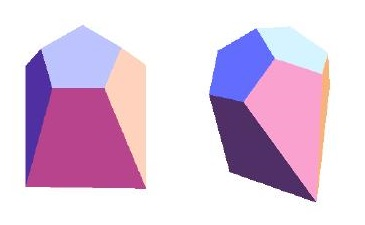Acronym ... Name cell of gap dual,adjacent bikis doe© Vertex figure , [52,K], [5,K2], [5,K,T], [k2,t2] Lace cityin approx. ASCII-art o o f f o o x x v f v v f x x o o Dihedral angles (at margins) at singular long edge:   arccos(1/sqrt(5)) = 63.434949° at all other edges:   arccos(-1/sqrt(5)) = 116.565051° Confer uniform relative: doe   gad

This polyhedron can also be obtained as a stellation of doe by blending at 2 neighbouring pentagons with tall pyramids, which are the tips of sissid and then inserting inbetween a wedge type simplex, as is used for gad.

The faces are regular pentagons of side v = (sqrt(5)-1)/2 = 0.618034; kites {(k,K,K,K)} with corner angle k = 36° and K = 108°, where side KK has size v again, diagonal KK has size x = 1, side kK has size f = (1+sqrt(5))/2 = 1.618034, and the diagonal kK has size sqrt[(5+sqrt(5))/2] = 1.902113; and trapzia {(t,t,T,T)} with corner angle t = 72° and T = 108°, where the side TT has size v again, while the other sides tT and tt have both size f again.

Incidence matrix according to Dynkin symbol

ofoxv fvfxo&#(f,v)t   → height(1,2) = (3+sqrt(5))/4 = 1.309017
height(2,3) = height(4,5) = (sqrt(5)-1)/4 = 0.309017
height(3,4) = (3-sqrt(5))/4 = 0.190983

o.... o....         | 2 * * * * | 1 2 1 0 0 0 0 0 | 2 2 0 0  [k2,t2]
.o... .o...         | * 4 * * * | 0 1 0 1 1 0 0 0 | 1 1 1 0  [5,K,T]
..o.. ..o..         | * * 2 * * | 0 0 1 0 0 2 0 0 | 0 2 0 1  [5,K2]
...o. ...o.         | * * * 4 * | 0 0 0 0 1 1 1 0 | 0 1 1 1  [52,K]
....o ....o         | * * * * 2 | 0 0 0 0 0 0 2 1 | 0 0 1 2  
--------------------+-----------+-----------------+--------
..... f....         | 2 0 0 0 0 | 1 * * * * * * * | 2 0 0 0
oo... oo...&#f      | 1 1 0 0 0 | * 4 * * * * * * | 1 1 0 0
o.o.. o.o..&#f      | 1 0 1 0 0 | * * 2 * * * * * | 0 2 0 0
..... .v...         | 0 2 0 0 0 | * * * 2 * * * * | 1 0 1 0
.o.o. .o.o.&#v      | 0 1 0 1 0 | * * * * 4 * * * | 0 1 1 0
..oo. ..oo.&#v      | 0 0 1 1 0 | * * * * * 4 * * | 0 1 0 1
...oo ...oo&#v      | 0 0 0 1 1 | * * * * * * 4 * | 0 0 1 1
....v .....         | 0 0 0 0 2 | * * * * * * * 1 | 0 0 0 2
--------------------+-----------+-----------------+--------
..... fv...&#f      | 2 2 0 0 0 | 1 2 0 1 0 0 0 0 | 2 * * *  {(t,t,T,T)}
oooo. oooo.&#r(f,v) | 1 1 1 1 0 | 0 1 1 0 1 1 0 0 | * 4 * *  {(k,K,K,K)}, cycle (a,b,d,c)
..... .v.xo&#vt     | 0 2 0 2 1 | 0 0 0 1 2 0 2 0 | * * 2 *  {5}
..oxv .....&#vt     | 0 0 1 2 2 | 0 0 0 0 0 2 2 1 | * * * 2  {5}